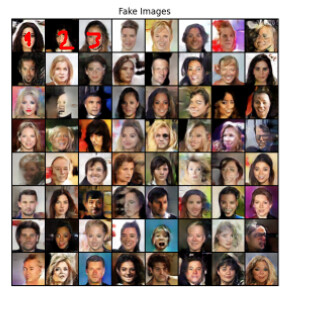# How do you save the images generated by a pytorch generative adversarial network?

I have followed this Pytorch tutorial on DCGAN to the letter, I managed to generate images successfully, however I cannot extract the generated images individually, they are only generated in the mosaic.Is there a way to save the generated images one by one?

The tutorial uses different approaches to visualize the entire grid:

``````fig = plt.figure(figsize=(8,8))
plt.axis("off")
ims = [[plt.imshow(np.transpose(i,(1,2,0)), animated=True)] for i in img_list]
ani = animation.ArtistAnimation(fig, ims, interval=1000, repeat_delay=1000, blit=True)

HTML(ani.to_jshtml())
``````

and

``````plt.imshow(np.transpose(vutils.make_grid(real_batch.to(device)[:64], padding=5, normalize=True).cpu(),(1,2,0)))
``````

If you want to visualize specific images alone, remove the list comprehension or the usage of `vutils.make_grid`.

1 Like